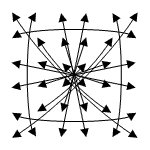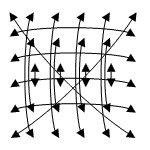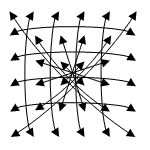Structure of Magic and Semi-Magic Squares, Methods and Tools for Enumeration by Francis GaspalouI remember there are no pandiagonal squares of order 6 (nor symmetrical). The number of magic squares of order 6 is still unknown; this number has been estimated (see Walter TRUMP's site).

 1. Orthogonal Squares to Latin Diagonal Squares

It is well known that there is no Greco-Latin square of order 6 (cf. EULER's problem of the 36 officers). Therefore,
I searched an intermediate square - necessarily not Greco-Latin - giving a maximum of squares, this maximum being inferior to that given by an hypothetic Greco-Latin square, that is to say 14 * (6!)2 = 7 257 600 (see The intermediate square method, § 4). I remember I don't apply the group G of transformations to this intermediate square.

I first searched Latin diagonal squares (they exist!), then I searched orthogonal squares to these Latin diagonal squares: I found such orthogonal squares by a program with 23 parameters! It seems that these squares - which are a specific set of semi-regular squares - form an unexplored mine!
Among these squares, I particularly studied squares with regular rows.

I give the results of my research. The method is the same as for orthogonal to Latin diagonal squares of order 5 (see Appendix 4, § 4).

1.1 Enumeration of Latin diagonal squares

I remember that a Latin diagonal square is a square with two Latin diagonals.

I searched elementary Latin diagonal squares with 1, 2, 3, 4, 5, 6 on the first diagonal and with A6< E1. I found 64 elementary solutions (program with 18 parameters). This number has to be multiplied by 2*(6!) = 1 440 to get the total number of solutions: there are 92 160 Latin diagonal squares of order 6.

If you apply the group G of order 192 and the 720 permutations (1 2 3 4 5 6), you will see that the 64 elementary solutions can be reduced to 2 (the first 2 among 64 for example):

 1 3 4 5 6 2 4 2 6 3 1 5 5 1 3 6 2 4 2 6 5 4 3 1 6 4 2 1 5 3 3 5 1 2 4 6
and
 1 3 4 5 6 2 5 2 6 3 1 4 4 1 3 6 2 5 2 6 5 4 3 1 6 4 2 1 5 3 3 5 1 2 4 6

These 2 squares come from a same elementary square (Latin only and not Latin diagonal): the BOUTELOUP's square type 12 or the TARRY's type 7 bis.

Then, by application of group G of 192 and permutations,
 the first square gives (192 * 720)/2 = 69 120 induced squares, the second square (192 * 720)/6 = 23 040 «            « total 92 160 Latin diagonal squares

(the first square is the same - after permutation - when you apply the transformation V,
and the second square is the same - still after permutation - when you apply V but also 4 other transformations making with V a group of order 6, subgroup of group G of 192).

1.2 Enumeration of orthogonal squares to Latin diagonal squares

Number of orthogonal squares to these 2 elementary squares: 90 164 and 76 764 given by my program with 23 parameters.

There are:
 90 164 * 69 120 = 6 232 135 680 + 76 764 * 23 040 = 1 768 642 560 8 000 778 240 orthogonal squares with the first basis and 8 000 778 240 * 7 = 56 005 447 680 if you consider the 14 basis (cf. explanation hereafter).

Note # 1: you can compare with orders 4 and 5

Order 4Order 5Order 6
Number of Latin
diagonal squares
48

960
(480+480)
92 160
(69 120 + 23 040)
Number of elementary
squares
122
Number of orthogonal
squares for each
24536 and 32090 164 and 76 764
Number of orthogonal
squares with the first basis
1 152410 8808 000 778 240
Number of orthogonal
squares with all the basis
3 456410 88056 005 447 680

For order 4, there are 3 456 orthogonal squares, which are semi-pandiagonal. These squares are obtained with the 3 first basis; the 3 last basis give the same squares (see Appendix 3, § 5).
As a matter of fact, if you have one magic square which is solution, this square generates one intermediate square with one basis. And with the corresponding basis, this intermediate square gives the same magic square if you permute the capital and the lower-case letter squares (see definition of the corresponding basis in The intermediate square method, § 3.2). Therefore, to get the total number of orthogonal squares, you have to multiply by k/2 - and not by k - the number of squares for one basis.

The notion of Latin is specific to a basis: a line is not Latin by itself; if you change the basis the property may disappear.

I suppose here that the equivalent set of 3 456 semi-pandiagonal squares of order 4 is for superior orders the set of orthogonal squares to Latin diagonal squares (with all the basis). But I haven't the relations of definition of such sets and I don't know if such relations exist.

Question: is it possible to give relations of definition for the sets of order 5 and 6?

Note # 2: you can take another definition for the equivalent set of 3 456 semi-pandiagonal squares of order 4. For
superior orders, you can suppose that it is the set of regular squares (with all the basis).

Order 4Order 5Order 6Order 7
Number of regular squares
(with all the basis)

3 456 (semi-pandiagonal)

57 600
(pandiagonal and one other isomorphic set)

0

2 438 553 600
(pandiagonal and 7 other isomorphic sets)

See explanations in The intermediate square method, § 4. For order 7, I am not absolutely sure that pandiagonal squares with their isomorphic sets are the only regular squares.

Note # 3: in Appendix 4, § 3, I suggest another definition of semi-pandiagonal squares of order 5 and I propose a
way to find relations of definition.

1.3 Sets generated by some intermediate squares

• Among the orthogonal squares to these two Latin diagonal squares, I studied some intermediate squares obtained with the classical basis (1,2,...,6;0,6,...,30) and the sets generated by these intermediate squares:

• sets which are the most often encountered: sets of 8 * 720 = 5 760 squares

Magic conditions: B-A=C-B=D-C=E-D=F-E on capital letters
no condition on lower-case letters naturally

Therefore: 4 possible basis among 14,
2 solutions per basis for capital letters,
6! = 720 solutions for lower-case letters,
total: 8 * 720 squares

Example:  A+a A+c A+d F+e F+f F+b B+d E+b E+f C+c B+a D+e E+e C+a F+c A+f C+b C+d D+b C+f A+e E+d D+c D+a D+f F+d B+b E+a B+e B+c E+c C+e F+a A+b D+d B+f

• sets of 32 * 720 = 23 040 squares are also encountered

• I particularly studied the orthogonal squares with regular rows (or columns; I computed all these orthogonal squares to the two elementary Latin diagonal squares)

• sets of 72 * 720 = 51 840 squares:

Example:  A+a B+d C+e E+b F+f D+c C+c B+b F+a D+f E+d A+e C+d E+f F+c B+e A+b D+a F+e E+c A+f D+d B+a C+b B+f C+a D+b A+c E+e F+d F+b D+e A+d E+a B+c C+f

Magic conditions: B-A=D-C=F-E
and C-B=E-D

Note: the 6 rows and the 2 diagonals are regular.

• sets of 144 * 720 = 103 680 squares

Example:  B+a A+e C+d F+b D+f E+c D+c E+b F+a A+f B+d C+e D+d F+f B+c E+e C+b A+a F+e C+c B+f E+d D+a A+b C+f E+a D+b A+c B+e F+d B+b A+d D+e C+a F+c E+f

Magic conditions: B-A=E-D
and B+E=Σ/3 (Σ=A+B+C+D+E)

Note: only the 6 rows are regular.

• record: sets of 14 * 48 * 720 = 483 840 squares

Example:  A+a B+d C+e D+b F+f E+c D+c F+b B+a C+f A+d E+e F+d E+f C+c B+e A+b D+a F+e A+c D+f E+d C+a B+b A+f F+a E+b B+c D+e C+d C+b A+e D+d E+a F+c B+f

Magic conditions: A+F=B+E=C+D

Note: the 6 rows and the 2 diagonals are regular.

Therefore, I found sets with more squares than the classical sets known as the "square of the sun":

 1 35 34 3 32 6 30 8 28 27 11 7 24 23 15 16 14 19 13 17 21 22 20 18 12 26 9 10 29 25 31 2 4 33 5 36

i.e.:  A+a B+d C+e D+b F+f E+c D+c F+b B+a C+f A+d E+e F+d E+f C+c B+e A+b D+a F+e A+c D+f E+d C+a B+b A+f F+a E+b B+c D+e C+d C+b A+e D+d E+a F+c B+f

Magic conditions: A+F=B+E=C+D
a+f=b+e=c+d

Set of 14 * 482 = 32 256 squares only.

The record of 483 840 squares is very inferior to the theoretical number of  7 257 600 given by an hypothetical Greco-Latin square.

• Questions:

 - is it possible to do better than 483 840? - in a square of order 6, I found for the moment a maximum of 8 regular "lines" among the6 rows + 6 columns + 2 diagonals = 14 possible lines. Is it possible to do better?

 2. Other Researches Top of the page• I searched magic squares of a given "type", as:There is no difficulty to make programs and to find solutions for these types, as well as for bordered squares.

The first pattern (program with 14 parameters) is very fruitful; you can deduce from it - with supplementary conditions - numerous other patterns, like the second.

• I searched "totally irregular" squares, i.e. squares with all the lines (rows, columns and 2 diagonals) which are irregular, but I didn't succeed.

You can see that the minimum order of a totally irregular square is 6. As a matter of fact, there is evidently 0 solution for order 4 (all the squares are known: only the 2 diagonals may be irregular) and you easily demonstrate it is impossible to find a solution for order 5.

Here is the demonstration. If you search a lower-case square of order 5 with irregular lines, the total of each line is 0 modulo 5, therefore the possible values for this total are 5, 10, 20 or 25 because 15 is excluded. The total of 5 rows is 5*15=75, and the 5 totals of rows are made with 10 and 20 and exceptionally with 5 (1+1+1+1+1) or 25 (5+5+5+5+5) which can appear only one time because there are only 5 numbers "1" and 5 numbers "5" in the square.
If one row has a total of 5 (1+1+1+1+1), then the other rows have a total of 10 or 20. But for columns, you have necessarily one column with a total or 5 or 25. 5 is impossible because all the numbers "1" are occupied and 25 is impossible too because you have necessarily one "1" in that column.
The demonstration is the same if one row has a total of 25.

Question: can anyone find a totally irregular square of order 6?